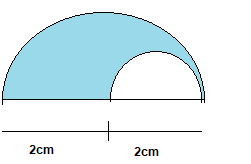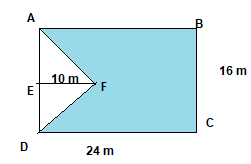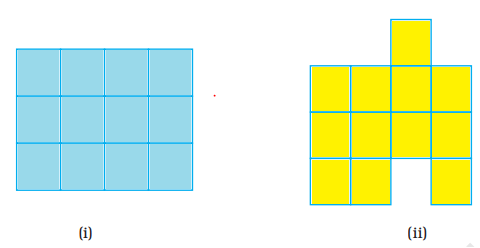# Perimeter and Area Important Questions

In this page we have Perimeter and Area Important Questions for Class 7 Maths Chapter 12 . Hope you like them and do not forget to like , social share and comment at the end of the page.
Question 1.
Fill in the blanks :
i. A figure which consists of line segments only is called a______________
ii.  Perimeter of a regular polygon is_____________________
iii. Perimeter of an irregular polygon is______________________
iv. Distance around a circle is called the____________________of the circle.
v. Ratio of circumference of the circle to its diameter is____________________ and named by the Greek letter__________________________.
vi. Two circles with same centre but different radii is called______________________
vii. A chord of a circle contains exactly___________________points on the circle.
ix. One hectare = _____________________ m2
x. 500 sq. m = _____________________sq. cm.
xi.If a wire in the shape of a square is rebent into a rectangle, then the _______ of both shapes remain same, but ______may varry
xii. The circumference of a circle whose area is  $81 \pi r^2$, is ________
Question 2.
The figure shows two semi-circles. Find the area of the shaded part in terms of  $\pi$Question  3.
The base of a parallelogram is thrice its height. If the area is 897 sq.cm. Find the base and height of the parallelogram.
Question 4.
The legs of a right triangle are in the ratio of 3:4 and its area is 1014 sq.cm. Find its hypotenuse.
Question 5.
The area of two circles are in the ratio 25:36. Find the ratio of their circumference.
Question 6.
From a rectangular metal sheet of sides 30 cm and 40 cm, a circular sheet as big as possible is cut off. Find the area of remaining sheet.
Question 7.
ABCD is a rectangle with dimensions 24m by 16m. AFE is a triangle such that $EF \perp AD$ and EF = 10m. Calculate the area of the shaded portion.Question 8.
True and False statement(a) area of (i) is the same as the area of (ii).
(b) Perimeter of (ii) is the same as (i).
(c) If (ii) is divided into squares of unit length, then its area is 13 unit squares.
(d) Perimeter of (ii) is 18 units

Question 9
Find the area enclosed by the below figureQuestion 10
1.
i. polygon
ii.number of sides multiply by measure of the size
iii. sum of the all the sides
iv. circumference
v. pi ,$\pi$
vi. concentric circle
vii. two
ix. 10000
x, 5000000
xi. Perimeter,area
xii. $18 \pi r$
2. $\frac {3\pi}{2}$
3.
3x and x
$3x \times x=867$
$3x^2=867$
$x^2=289$
x=17
therefore height is 17m and base is 51m
4.
Let it's leg be 3x and 4x. Then,
according to question,
Area of right angle triangle = $\frac {1}{2} BH= \frac {1}{2} \times 3x \times 4x$
$1014 = 6x^2$
x=13 cm
Hence, first side = 3×13 = 39 cm
second side = 4×13 = 52 cm.
We know that  in right angle triangle
$Hyp^2 = Base^2 + height^2$
H = 65 cm.
Hence, the hypotenuse is 65 cm.
5 5/6
6.
The maximum circle which can be cut from rectangular sheet is decided by the lowest side. In this case ,it is 30 cm
So, Diameter of circular sheet = 30 cm
radius of circular sheet = 15 cm
Area of remaining sheet = Area of rectangular sheet - Circular sheet
$= L \times B - \pi r^2$
Substituting the values
Area of remaining sheet = $492.86 cm^2$
7.  Area of triangle AFE = $\frac {1}{2} \times 16 \times 10=80 m^2$
Area of the rectangle = $24 \times 16=384 m^2$
Area of the shaded portion = $384 -80 =304 m^2$
8.
a. True
b. false
c. false
d. true
9.46.45 sq cm

• Worksheets